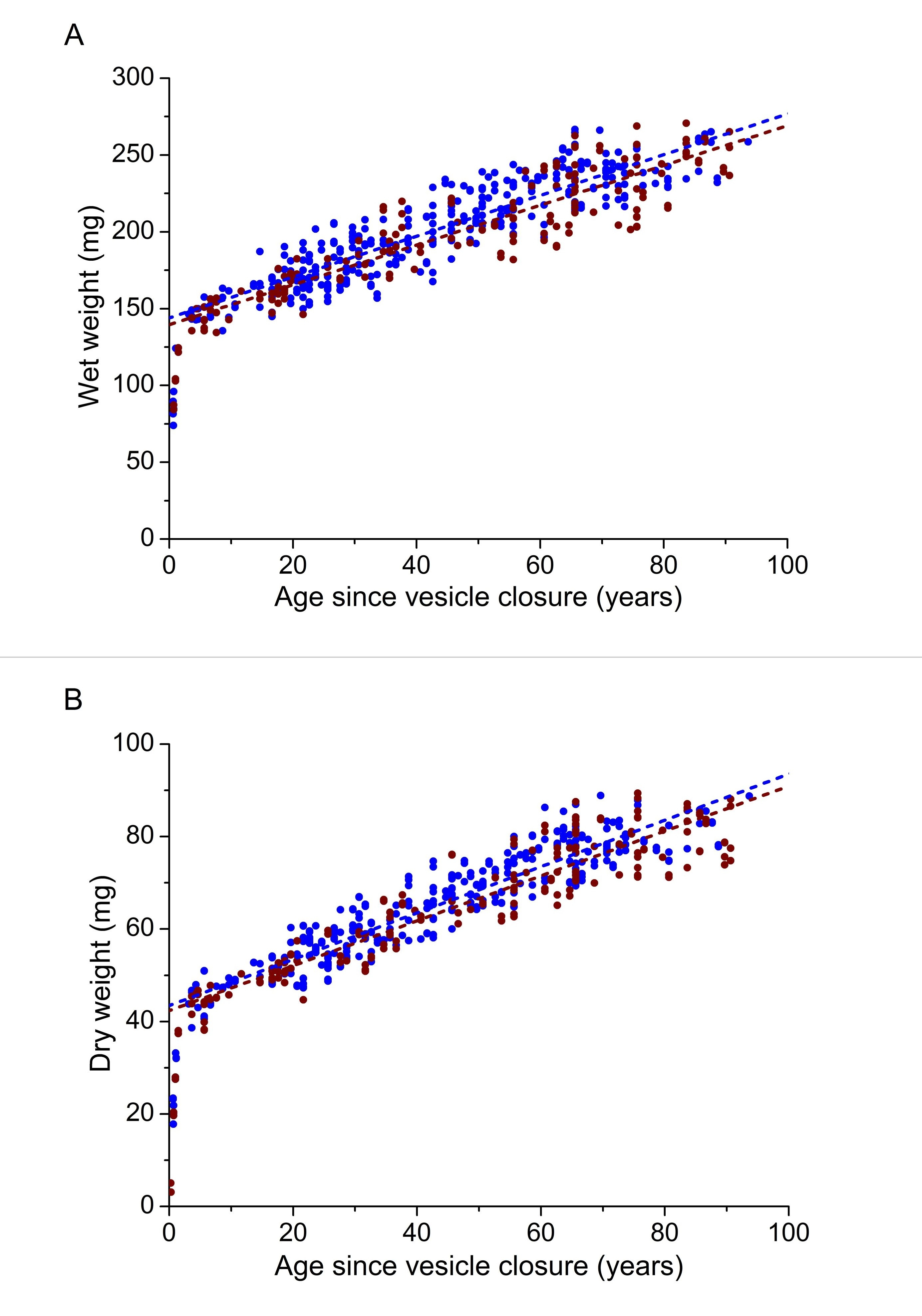Figure 5 of Mohamed, Mol Vis 2018; 24:867-874.

Figure 5. Human lens wet (A) and dry (B) weights as a function of age since vesicle closure for women (brown symbols) and men (blue symbols). Linear regression analyses of the 3- to 93-year-old data yielded the following relationships: female wet weight = 139.51 (±3.14) + 1.30 (±0.06) × Ab (R2=0.84;p<0.0001; n=96); female dry weight = 42.36 (±1.05) + 0.49 (±0.02) × Ab (R2=0.87; p<0.0001;n=100); male wet weight = 143.97 (±2.14) + 1.33 (±0.05) × Ab (R2=0.82; p<0.0001; n=199); male dry weight = 43.44 (±0.66) + 0.50 (±0.01) × Ab (R2=0.87; p<0.0001; n=202).